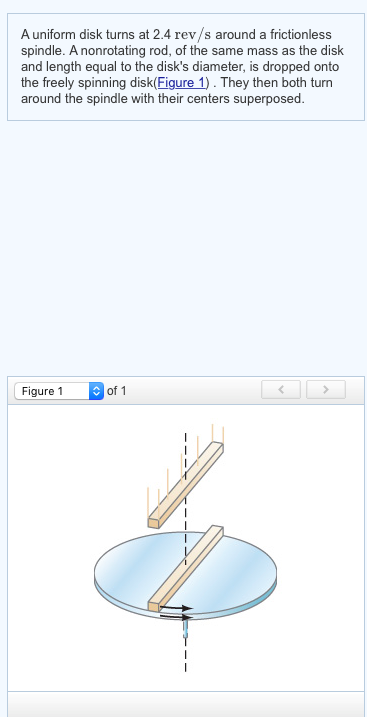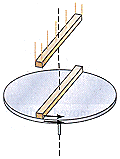# A uniform disk turns at 3.7 rev/s around a frictionless spindle. A nonrotating rod, of the same mass as the disk and len...

A uniform disk turns at 3.7 rev/s around a frictionless spindle. A nonrotating rod, of the same mass as the disk and length equal to the disk's diameter, is dropped onto the freely spinning disk. They then turn together around the spindle with their centers superposed. What is the angular frequency in rev/s of the combination?

Concepts and reason

The concepts used to solve this problem are law of conservation of angular momentum and the moment of inertia.

First, determine the expressions for the moment of inertia of the disk and the system of the disk and the rod. Finally, calculate the angular frequency of the system of disk and the rod by using the law of conservation of angular momentum.

Fundamentals

The angular momentum of the disk is,

${L_1} = {I_1}{\omega _1}$

Here, ${I_1}$is the moment of inertia of the disk and ${\omega _1}$is the angular speed of the disk.

The angular momentum of the system of disk and the rod is,

${L_2} = {I_2}{\omega _2}$

Here, ${I_2}$is the moment of inertia of the system of disk and rod and ${\omega _2}$is the angular speed of the system of disk and rod.

The moment of inertia ${I_{{\rm{disk}}}}$of a disk of radius $\ell$ about an axis passes through its centre is given by following expression.

${I_{{\rm{disk}}}} = \frac{1}{2}m{\ell ^2}$

The moment of inertia ${I_{{\rm{rod }}}}$of a rod of length $\ell$about an axis passes through its centre is given by following expression.

${I_{{\rm{rod}}}} = \frac{1}{{12}}m{\ell ^2}$

The moment of inertia of the disk is,

${I_1} = \frac{1}{2}m{\ell ^2}$

Here, $m$is the mass of the disk and $\ell$is the radius of the disk.

Given that, the length of the rod is equal to the radius $\left( \ell \right)$of the disk. So, the length of the rod is$2\ell$.

The moment of inertia of the system of disk and rod is,

$\begin{array}{c}\\{I_2} = {I_{{\rm{disk}}}} + {I_{{\rm{rod}}}}\\\\ = \frac{1}{2}m{\ell ^2} + \frac{1}{{12}}m{\left( {2\ell } \right)^2}\\\\ = \frac{1}{2}m{\ell ^2} + \frac{1}{3}m{\ell ^2}\\\end{array}$

From the law of conservation of angular momentum, the total initial angular momentum of the system of rod and disk is equal to the total final angular momentum of the system of rod and disk.

${I_1}{\omega _1} = {I_2}{\omega _2}$

Substitute$\frac{1}{2}m{\ell ^2}$for${I_1}$and $\frac{1}{2}m{\ell ^2} + \frac{1}{3}m{\ell ^2}$for${I_2}$.

$\begin{array}{c}\\\left( {\frac{1}{2}m{\ell ^2}} \right){\omega _1} = \left( {\frac{1}{2}m{\ell ^2} + \frac{1}{3}m{\ell ^2}} \right){\omega _2}\\\\\left( {\frac{1}{2}} \right){\omega _1} = \left( {\frac{1}{2} + \frac{1}{3}} \right){\omega _2}\\\\{\omega _1} = \left( {1 + \frac{2}{3}} \right){\omega _2}\\\\ = \frac{5}{3}{\omega _2}\\\end{array}$

Rearrange the above equation for${\omega _2}$.

${\omega _2} = \frac{3}{5}{\omega _1}$

Substitute $3.7{\rm{ rev/s}}$for${\omega _1}$.

$\begin{array}{c}\\{\omega _2} = \frac{3}{5}\left( {3.7{\rm{ rev/s}}} \right)\\\\ = 2.22{\rm{ rev/s}}\\\end{array}$

Ans:

The angular frequency of the system of disk and the rod is${\bf{2}}{\bf{.22 rev/s}}$.

##### Add Answer of: A uniform disk turns at 3.7 rev/s around a frictionless spindle. A nonrotating rod, of the same mass as the disk and len...
Similar Homework Help Questions
• ### A uniform disk turns at 2.7 rev/s around a frictionless central axis. A nonrotating rod, of the same mass as the disk a...

A uniform disk turns at 2.7 rev/s around a frictionless central axis. A nonrotating rod, of the same mass as the disk and length equal to the disk's diameter, is dropped onto the freely spinning disk(Figure 1) . They then turn around the spindle with their centers superposed. What is the angular frequency in rev/s of the combination?

• ### A uniform disk turns at 3.6 rev/s around a frictionless central axis. A nonrotating rod, of the same mass as the dis...

A uniform disk turns at 3.6 rev/s around a frictionless central axis. A nonrotating rod, of the same mass as the disk and length equal to the disk's diameter, is dropped onto the freely spinning disk(Figure 1). They then turn around the spindle with their centers superposed. Figure 1 of 1 Part A What is the angular frequency in rev/s of the combination? # - - - -

• ### A uniform disk turns at 3.6 rev/s around a frictionless spindle. A non rotating rod, of the same mass as the disk and l...

A uniform disk turns at 3.6 rev/s around a frictionless spindle. A non rotating rod, of the same mass as the disk and length equal to the disk's diameter, is dropped onto the freely spinning disk . They then both turn around the spindle with their centers superposed. What is the angular frequency in rev/s of the combination? please express answer in proper significant figures and rounding.

• ### What is the angular frequency in rev/s of the combination? A uniform disk turns at 2.4 rev/s around a frictionless sp...What is the angular frequency in rev/s of the combination? A uniform disk turns at 2.4 rev/s around a frictionless spindle. A nonrotating rod, of the same mass as the disk and length equal to the disk's diameter, is dropped onto the freely spinning disk(Figure 1). They then both turn around the spindle with their centers superposed. Figure 1 of 1

• ### Angular Frequency problem

A uniform disk turns at 3.7 rev/s around a frictionless spindle. A nonrotating rod, of the same mass as the disk and length equal to the disk's diameter, is droppedonto the freely spinning disk. They then turn together around the spindle with their centers superposed. What is the angular frequency in rev/s of the combination?

• ### angular frequencyA uniform disk turnsat 3.6 around a frictionless spindle. A nonrotating rod, of thesame mass as the disk and length equal to the disk's diameter, isdropped onto the freely spinning disk.They then turn togetheraround the spindle with their centers superposed.(Intro 1 figure)What is the angular frequency in of the combination?(Please explain well, thanks)

• ### physics

A uniform disk turns at 1.8 around a frictionless spindle. A nonrotating rod, of the same mass as the disk and length equal to the disk's diameter, is dropped onto thefreely spinning disk . They then both turn around the spindle with their centers superposed

• ### Rotational MotionA uniform disk turns at 9.5 rev/saround a frictionless spindle. A nonrotating rod, of the same massas the disk and length equal to the disk's diameter, is droppedontothe freely spinning disk, see the figure. They then turntogether around the spindle with their centers superposed. What isthe angular velocity of thecombination? rev/sHelp!

• ### Vectors/cross product/angular frequency / conservation of angular momentum.

i)The vector cross product of two vectors A and B is zero. What is the angle between these vectors?Show work.ii)A uniform disk turns at 2.4 rev/sec around a frictionless spindle. A nonrotating rod,of the same mass as thedisk and length equal to the disk diameter is dropped onto the freely spinning disk as shown in the figure.They then both turn around the spindle with theircenters superposed. What is the angular frequency in rev/sec of the combination? {Disk= I=(1/2)MR^2} and {Rod=I=(1/12)ML^2....

• ### Rotational Motion

A uniform disk turns at 5.0 rev/s around a frictionlessspindle. A nonrotating rod, of the same mass as the disk and lengthequal to the disk's diameter, is droppedonto the freely spinningdisk. They then both turn around the spindle with their centerssuperposed. What is the angular frequency in rev/s of thecombination?

Need Online Homework Help?